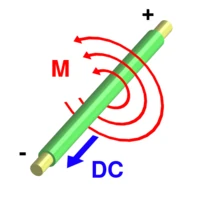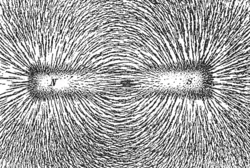Current flowing through a wire produces a magnetic field (M) around the wire. The field is oriented according to the right-hand rule.

In physics, a magnetic field is an entity produced by moving electric charges (electric currents) that exerts a force on other moving charges.

## Explained

(The quantum mechanics|quantum-mechanical  spin  of a particle produces magnetic fields and is acted on by them as though it were a current; this accounts for the fields produced by "permanent" Ferromagnetism .) A magnetic field is a vector field : it associates with every point in space a (pseudovector -)vector (spatial)  that may vary in time. The direction of the field is the equilibrium direction of a compass needle placed in the field.

## Symbols and terminology

Magnetic field is usually denoted by the symbol. Historically,was called the magnetic flux density  or magnetic induction. A distinct quantity,, was called the magnetic field, and this terminology is still often used to distinguish the two in the context of magnetic materials (non-trivial permeability μ). Otherwise, however, this distinction is often ignored, and both quantities are frequently referred to as "the magnetic field." (Some authors call H the auxiliary field, instead.) In linear materials, such as air or free space, the two quantities are linearly related:whereis the magnetic permeability (in henries per meter) of the medium.

In SI units,andare measured in teslas (T) and amperes per meter (A/m), respectively; or, in cgs units, in gauss (G) and oersteds (Oe), respectively. Two parallel wires carrying an electric current in the same sense will generate a magnetic field which will cause a force of attraction to each other. This fact is used to generate the value of an ampere of electric current. Note that while like charges repel and unlike ones attract, the opposite holds for currents: if the current in one of the two parallel wires is reversed, the two will repel.

## Definition

Like the electric field, the magnetic field can be defined by the force it produces. In SI units, this is:where

F is the force produced, measured in newtonsindicates a vector cross productis electric charge that the magnetic field is acting on, measured in coulombsis velocity of the electric charge, measured in metres per second
B is magnetic flux density, measured in teslas

This law is called the Lorentz force law . (More precisely, it is the special case of that law when there is no electric field. It holds in any reference frame , although the force due to the magnetic field may be different in different frames because magnetic fields transform into electric fields under Lorentz transformations . The total force due to the electric and magnetic fields is govened by the Lorentz transformation.)

### Current loop

A simpler form of the force equation in a wire current loop is:where

F = force (newton)
B = flux density (tesla)
L = length of wire (metre)
i = current in wire (ampere)

A more complex explanation is that if the moving charge is part of a current in a wire, then an equivalent form of the law isIn words, this equation says that the force per unit length of wire is the cross product of the current vector and the magnetic field. In the equation above, the current vector,, is a vector with magnitude equal to the usual scalar current,, and direction pointing along the wire that the current is flowing.

### Point charge generating magnetic field

The field can be computed as the sum of the contributions from individual charged particles. The magnetic flux density from a point charge is:which, for constant velocities, can be expanded into the Biot-Savart law :is electric charge generating the magnetic field, measured in coulombsis velocity of the electric chargethat is generating B, measured in metres per second
B is magnetic flux density, measured in teslas

## Properties

Changing magnetic fields, according to Faraday's law of induction , can induce an electric field and thus an electric current; similar currents can be induced by conductors moving in a fixed magnetic field. These phenomena are the basis for many electric generators and electric motors. See also 

### Magnetic field linesMagnetic field lines emanate primarily from the north pole of a magnet and curve around to the south pole. They can be revealed by scattering iron filings on a piece of paper over a magnet.

### Pole labeling confusions

It is necessary to note that the labeling of north and south on a compass is in opposition to the labeling of the north and south pole of the Earth.

If you have two labeled magnets, it is clear that like poles repel, while opposing poles attract. However, this is clearly wrong when using a compass to find the North Pole of the Earth, because the "north" end of the compass points to the "North" Pole.

By convention, the pole of a magnet is labelled according to the direction it points, hence when we speak of the "north pole" of a magnet, we really mean the "north-seeking pole". Magnetic field lines point from north to south of a magnet, and hence the natural magnetic field lines run from south to north along the Earth's surface. This choice, along with the choice of sign convention in the Biot-Savart law, is equivalent to choosing a sign convention for electric charge.

## Rotating magnetic fields

A rotating magnetic field is a magnetic field which rotates in polarity at non-relativistic speeds. This is a key principle to the operation of alternating-current motor. A permanent magnet in such a field will rotate so as to maintain its alignment with the external field. This effect is utilised in alternating current electric motors. A good rotating magnetic field can be constructed using three phase alternating currents (or even with higher order polyphase systems). Synchronous motors and induction motors use a stator's rotating magnetic fields to turn rotors.

 This page uses Creative Commons Licensed content from Wikipedia (view authors).